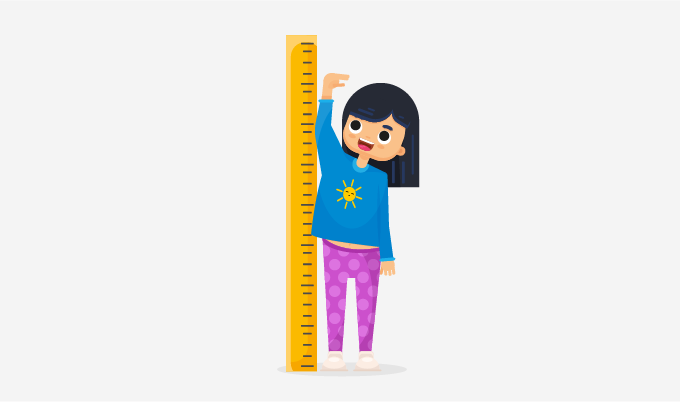# Round To The Nearest Tens - Definition with Examples

The Complete K-5 Math Learning Program Built for Your Child

• 40 Million Kids

Loved by kids and parent worldwide

• 50,000 Schools

Trusted by teachers across schools

• Comprehensive Curriculum

Aligned to Common Core

## Rounding

Rounding refers to the process of converting a particular number to an easy number. The rounded number is not exact but an estimate of the given number.

## Purpose of rounding

Usually, we round numbers for:

1. Making calculations easy.
2. Making the numbers easy to understand and remember.

## Applications of roundingEstimation: We use rounding to guess the most appropriate answer. Rounding is the process of estimation.

For example, Samantha wants to buy chocolates for \$97. She can estimate the amount to the nearest tens and give \$100. The shopkeeper will return Samantha \$3.

Estimation helps us to calculate easily.

## Rounding Numbers to the nearest tens

We can follow the given steps to round numbers to the nearest tens:

Step 1- We look at the number we want to round.

Step 2- As we are rounding the number to the nearest tens, we mark the digit in the tens column.

Step 3- Now we look at the ‘ones’ column (the digit to the right of the tens column).

Step 4- (i) If the digit in the one's column is 0, 1, 2, 3 or 4, we will round down the tens column to the nearest tens.

(ii) If the digit in the one's column is 5, 6, 7, 8 or 9, we will round up the tens column to the nearest tens.

Step 5- Write ones digit as 0 and remove all the digits after the one's column. The left out number is our desired answer.

Example 1: Round 524 to the nearest ten.

 Step Number Observation Working Out Step 1 524 Step 2 We get 2 in the tens column. Step 3 We get 4 in the one's column (right of 2) Step 4 We get 4 in Step 3. Round-Down Step 5 520 We keep 2 as it is and write ones digit as 0 and remove all the digits from the right of the one's column.

Example 2: Round 117.32 to the nearest tens.

 Step Number Observation Working Out Step 1 117.32 Step 2 We get 2 in the tens column. Step 3 We get 7 in the one's column (right of 1) Step 4 We get 7 in Step 3. Round-Up Step 5 120 We add 1 to the 1 at tens place and write one's digit as 0 and remove all the digits from the right of the one's column.

Example 3: Tom is 149 cm tall. Write his height in the nearest tens?Step Number Observation Working Out Step 1 149 Step 2 We get 4 in the tens column. Step 3 We get 9 in the one's column (right of 4) Step 4 We get 9 in Step 3. Round-Up Step 5 150 We add 1 to 4 at the tens place and write one's digit as 0 and remove all the digits from the right of the ones column

Exception case:

Example 4: Round the number 199.278 to the nearest tens.

 Step Number Observation Working Out Step 1 199.278 Step 2 We get 9 in the tens column. Step 3 We get 9 in the one's column (right of 9) Step 4 We get 9 in Step 3. Round-Up Step 5 200 We add 1 to 9 and carry 1 to hundreds column and write one's digit as 0 and remove all the digits from the right of the one's column.

Example 5: A park measures 249.9 meters in length. How long is the park to the nearest 10 meters?Step Number Observation Working Out Step 1 249.9 Step 2 We get 4 in the tens column. Step 3 We get 9 in the one's column (right of 4) Step 4 We get 9 in Step 3. Round-Up Step 5 250 We add 1 to 4 and write ones digit as 0 and remove all the digits from the right of the ones column.

 Fun Fact Around the world, a few retail outlets set their prices like \$99, \$199, \$0.99, \$9.99, etc. This is done because generally, people read from left to right, resulting in the first number striking in our head. Because of this, we tend to think that the prices are cheaper.

Won Numerous Awards & Honors